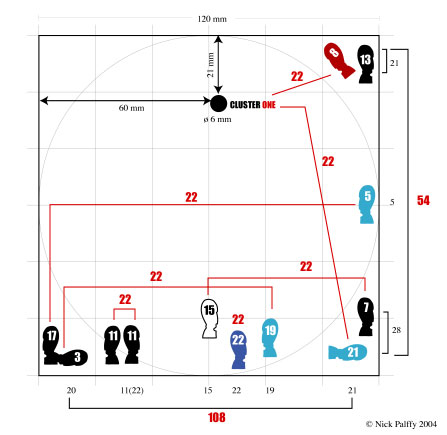# Significance of Numbers 54 and 108

Two very important numbers show up when we total the numbers by row and column. The sum of the right column amounts to 54. The sum of the bottom line number totals 108. It is certain that these numbers were intentionally designed into the booklet. The perfect 1/2 or 2/1 ratio cannot be accidental. Moreover, these are SACRED NUMBERS !More intriguing, the width of the open booklet (240 mm), when multiplied by 108, give the fascinating number of 25920, which is, incidentally, the equivalent of ONE Precessional Year (240 x 108 = 25920 years). Half of a precessional year amounts to 25920 / 2 = 12960 years, a figure easily obtained (54 x 240) in the booklet !

What does it mean?

It means that we're talking about Astronomy, the measure of Time, and.. The Division Bell!

This, again leads us to the division of the circle in 360°.

432 (4 x 108) = The Consecration.
432 x 60 = 216 x 120 = 108 x 240 = 54 x 480 = 27 x 960 = 25920

And 25920 = 72 x 360.
72 + 36 = 90 + 18 = 108.
360 / 108 = 3.3333.
360 / 54 = 6.6666.

First conclusion: The Booklet contains clues to COSMOLOGICAL KNOWLEDGE. This knowledge is strongly related to the DIVISION OF TIME by means of NUMBERS. The Division Bell has to do with MATHEMATICS, ASTRONOMY and GEOGRAPHY.

This means that these numbers must be related to SACRED SITES, such as the PYRAMIDS or STONEHENGE.

The literature that can be found on the Web is just too numerous to be cited here. But just have a look at MARTIN DOUTRE WEBSITE for a glimpse of the richness of these numbers and the tremendous dimensions they open...

"The number 54 was the numerical key to unlocking dynamic ratio and mathematical progression codes residing in all the standards of each ancient Mediterranean nation" . (http://www.celticnz.co.nz/Weights_Measures_Volumes/Weights_Measures.htm)

## Some numbers to crunch...

72 + 36 = 108

108 x 2 = 216

216/22 = 9.818181 (Newton's constant of gravity)